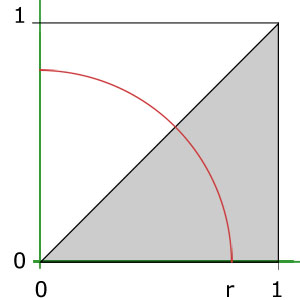BrainDen.com - Brain Teasers
• 0

## Question

A triangle has sides of length {1, 1, sqrt(2)}.

What is the length of the shortest cut that divides the triangle into two pieces that have equal areas?

## Recommended Posts

• 0

I'll take a quick stab at it:

Spoiler

sqrt(2π) / 4 = ~0.62666

The "obvious" first answer is sqrt(2) / 2 (bisect the 90 degree angle perpendicular to the hypotenuse)...But if we step back and look at it a different way: The current triangle has an area of 1/2...

So, we simply need to make a cut that leaves two areas of 1/4 (not necessarily similar shapes). The shortest STRAIGHT cut would be the sqrt(2) / 2, but if we decide to make a curved cut, we can get it shorter. I'll try to describe it (can't add pictures right now)

Choose one of the 45 degree angle points and center a circle there with radius r. Mark the points along the hypotenuse and adjacent leg at points to create a segment of a circle with a 45 degree angle and radius r. We know, then, that is exactly 1/8 of the area of the full circle. Since we are trying to get an area of 1/4, and we have 1/8 of a circle, the full area of the circle would be 2. so we can figure out the length of r by doing πr2=2. Leaving us with r = sqrt(2π) / π

Given that, we need to find the length of just that arc of the circle...which is 1/8 of the perimeter. So we know P=πd...which leaves us with P=2*sqrt(2π). Divide it by 8, and you get sqrt(2π) / 4 as the length of the arc connecting your points...leaving 2 pieces of the triangle with areas of 1/4 each.

Edited by Pickett
It duplicated my response for some reason....
##### Share on other sites

• 0

Nice stab!

I made a picture of it.

Spoiler## Join the conversation

You can post now and register later. If you have an account, sign in now to post with your account.×   Pasted as rich text.   Paste as plain text instead

Only 75 emoji are allowed.

×   Your previous content has been restored.   Clear editor

×   You cannot paste images directly. Upload or insert images from URL.